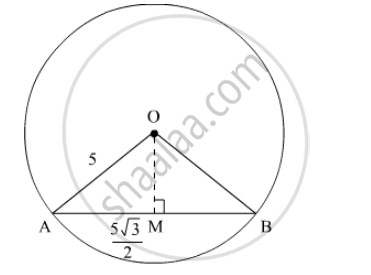Advertisement Remove all ads

# If Ab is a Chord of Length 5 √ 3 Cm of a Circle with Centre O and Radius 5 Cm, Then Area of Sector Oab is - Mathematics

MCQ

If AB is a chord of length $5\sqrt{3}$ cm of a circle with centre O and radius 5 cm, then area of sector OAB is

#### Options

• $\frac{3\pi}{8}c m^2$

• $\frac{8\pi}{3}c m^2$

• $25 \pi cm^2$

• $\frac{25\pi}{3}c m^2$

Advertisement Remove all ads

#### Solution

We have to find the area of the sector OAB.We have,

AM=(5sqrt3)/2

So,

sin ∠ AOM=(5sqrt3)/(2(5))

Hence,

∠ AOM=60°

Therefore area of the sector,

=1/2r^2θ

=1/2 (25)(2pi/3)

=(25pi)/3 cm^2

Is there an error in this question or solution?
Advertisement Remove all ads

#### APPEARS IN

RD Sharma Class 10 Maths
Chapter 13 Areas Related to Circles
Q 27 | Page 71
Advertisement Remove all ads

#### Video TutorialsVIEW ALL 

Advertisement Remove all ads
Share
Notifications

View all notifications

Forgot password?
Course